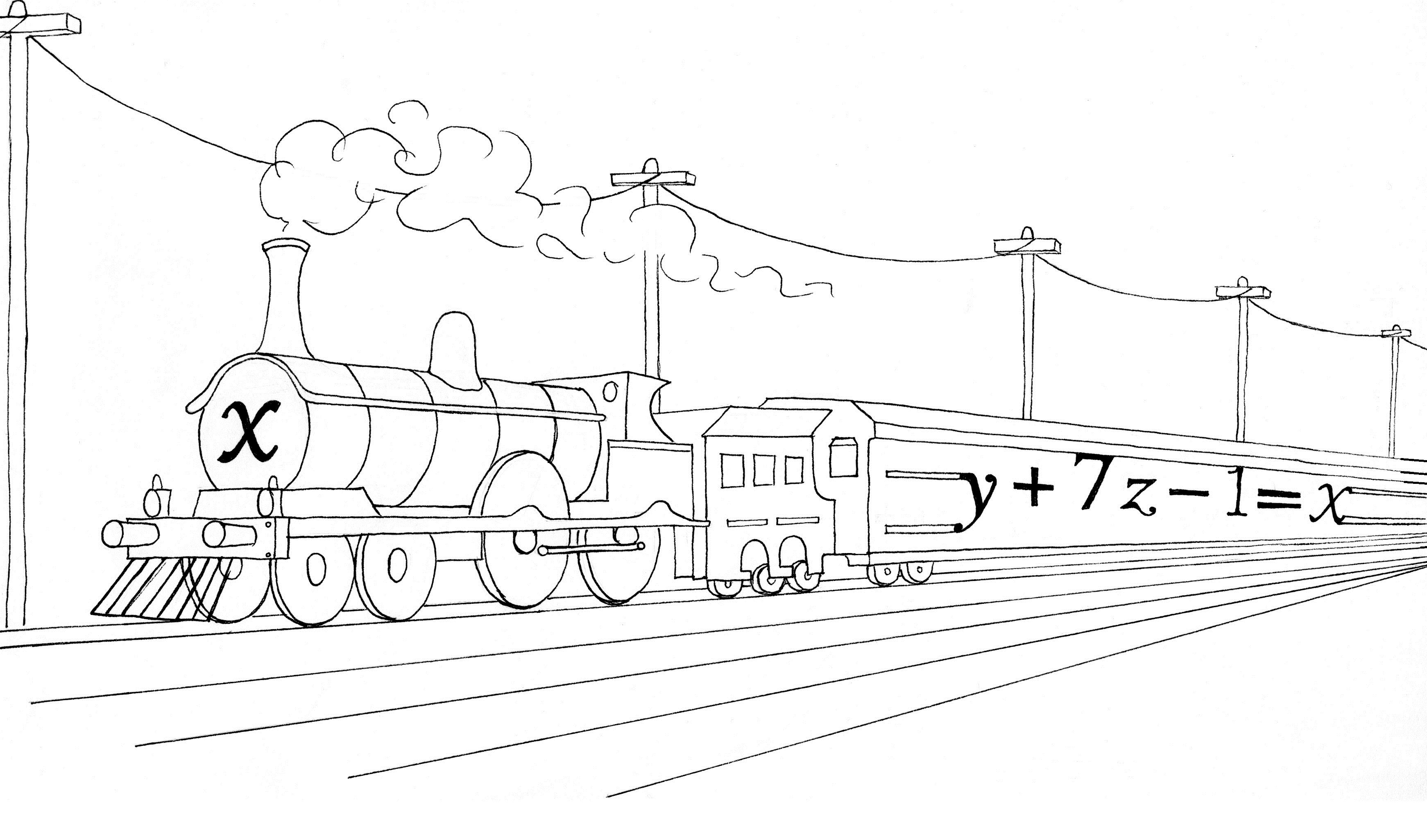SciTech

# Carnegie Mellon faculty improve algorithm to solve equationsCredit: Adelaide Cole/Assistant Art Editor

Linear equations are a fundamental part of mathematics. Many of us have been taught how to solve these equations for an unknown variable since middle school. In the real world, applications of linear equations — or, in some cases, systems of linear equations — are significant. Unfortunately, solving a large set of linear equations can be time-consuming for algorithmic solvers, but researchers from Carnegie Mellon’s School of Computer Science have developed an algorithm that uses graph theory and linear algebra to expedite the solving process.

Gary Miller, a faculty member in the School of Computer Science, teaches both undergraduate and graduate courses in algorithms, along with graduate courses in computational geometry and spectral graph theory. He started his research on linear equations in the 1990s. He called the traditional method of solving linear equations, known as Gaussian elimination, “both space-inefficient and time-inefficient,” when it comes to large sets of linear equations.

“For [linear equations] problems these days, it’s not unusual to have a billion variables,” Miller said from his office in the Gates Hillman Complex. “This is a classic problem in theoretical computer science.”

Gaussian elimination is a method commonly presented in junior high and high school in which systems of multivariable linear equations are solved by eliminating one of the variables and solving in terms of another. While this method has use for problems relating just a few variables, a greater number of variables would increase the time required to solve the equations manually. According to an article on Dr. Dobb’s Journal, a computer programming website, the time it takes for a computer to solve a system of linear equations is proportional to the cube of the number of variables.

Miller, systems scientist Ionnis Kurtis, and graduate student Richard Peng applied graph theory and a class of problems known as symmetric diagonally dominant (SDD) systems to linear systems. The same information that can be modeled in a graph using graph theory can also be modeled in a matrix with SDD systems. These matrices typically require a lot of time to solve, but with the new algorithm, such a matrix can be solved in a matter of seconds. In the new algorithm, the data is first imported into a matrix, which generates a sequence of graphs that can be solved using the data and a method known as Chebyshev iteration.

The same Dr. Dobb’s article states that the new algorithm’s run time, for a system of n variables, is proportional to n[log(n)]2, almost a million times faster than Gaussian elimination.

Real-world systems of linear equations can take many forms. Miller used a circuit diagram of resistors and wires as an example. The diagram is considered the weighted graph, and the voltage and resistance values in the circuit can be modeled in a matrix. The algorithm can be applied to the matrix for large circuits to solve for any unknowns. Miller also used the Netflix movie rental website, which uses trend analyses for viewers to suggest similar movies to ones that they have already watched, as another example.

As for marketing the code, Miller reported a fair number of users using the code with success. The University of Pittsburgh Medical Center, for example, has used similar coding in the past for biological retinal images of the eye and of cartilage of the knee. Miller also encouraged other programmers to distribute and debug their code for testing. “You’re allowed to have the whole world help you develop this great piece of code,” Miller said. “And then at some later date, you can then sell it to someone.”

“What I’m excited about is the [applications of the algorithm] that I haven’t thought of,” he said. “By releasing the code, people hopefully will tell us a bunch of new [applications].”